Courses

# Electrostatics -1

## 10 Questions MCQ Test Topicwise Question Bank for Electronics Engineering | Electrostatics -1

Description
This mock test of Electrostatics -1 for Electrical Engineering (EE) helps you for every Electrical Engineering (EE) entrance exam. This contains 10 Multiple Choice Questions for Electrical Engineering (EE) Electrostatics -1 (mcq) to study with solutions a complete question bank. The solved questions answers in this Electrostatics -1 quiz give you a good mix of easy questions and tough questions. Electrical Engineering (EE) students definitely take this Electrostatics -1 exercise for a better result in the exam. You can find other Electrostatics -1 extra questions, long questions & short questions for Electrical Engineering (EE) on EduRev as well by searching above.
QUESTION: 1

### What is the ratio of electrostatic repulsion and gravitational attraction between two electrons? Given: Q = Charge on an electron = 1.6 x 10-19C G = Gravitation constant = 6.67 x 10-11 Nm2/kg2 m = Mass of an electron = 9.1 x 10-31 kg

Solution:

By columb’s law,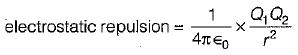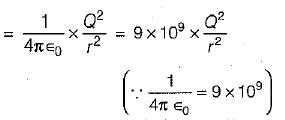Also, by Newton's law of gravitation,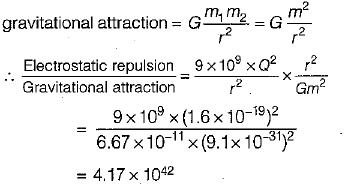QUESTION: 2

### What is the electric field strength at a distance of 200 mm from a charge of 2 x 10-6 Coulomb, in vacuum?

Solution: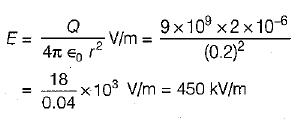QUESTION: 3

### Two point charges of 3 x 10-9 C and -2 x 10-9C are spaced two meters apart. What is the electric field at a point which is one meter from each of the two point charges?

Solution: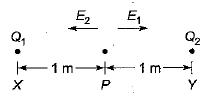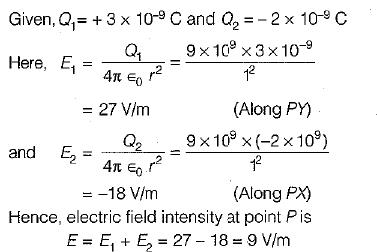QUESTION: 4

Five equal point charges of Q = 20 x 10-9C, are placed at x = 2,3,4,5 and 6 cm. The electric potential at origin will be

Solution:

Electric potential at origin is given as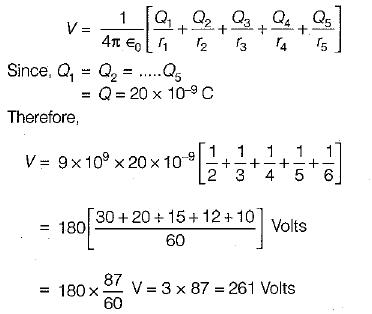QUESTION: 5

What is the equivalent capacitance of a parallel plate capacitor having two dielectrics, ∈r1 = 1.5 and ∈r2 = 3.5, each comprising one-half the volume as shown in figure below with A = 2 m2 and d = 1 mm?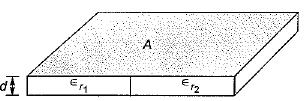Solution: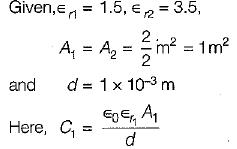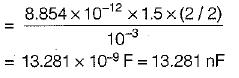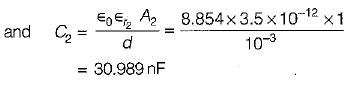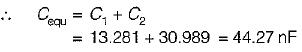QUESTION: 6

Four point charges 1, - 2, -3 and 4 μC are located on the x-axis at x = 1, 2, 3 and 4 metre respectively. The energy stored in the field is

Solution: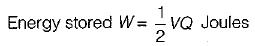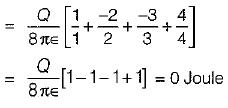QUESTION: 7

The electric field strength at a point in front of an infinite sheet of charge is

Solution:

The electric field strength at a point in front of an infinite sheet of charge is given by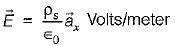where, ρs = charge density and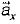= unit vector normal to the sheet and directed away from the sheet.
Here,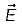is independent of the distance of the point from the sheet.

QUESTION: 8

Assertion (A): If all the points in space which have the same potential are jointed, then equipotential surfaces are obtained.
Reason (R): The field and the equipotential surfaces are orthogonal to each other.

Solution:

Both assertion and reason are individually true. However, the correct reason for assertion is that equipotential surfaces are those on which the potential is everywhere the same. Thus, if all the points in space which have the same potential are joined, then equipotential surfaces are obtained.

QUESTION: 9

Consider the following statements associated with various medias encountered in electostatics:
1. An isotropic media is one whose properties are independent of direction
2. A homogeneous media is one whose physical characteristics vary from point-to- point.
3. A non-homogenous media is one whose physical characteristics do not vary from point-to-point:
4. A linear media is a one in which the electric flux density is proportional to the electric field intensity.

Which of the above statements are correct?

Solution:

Statements 2 and 3 are not correct because a homogenous media is one whose physical characteristics do not vary from point-to-point while a non-homogeneous media is one whose physical characteristics vary from point-to-point.

QUESTION: 10

Assertion (A): Continuity of current equation is derived from law of conservation of charge.
Reason (R): Continuity of current equation states that there can be no accumulation of charge at any point.

Solution: